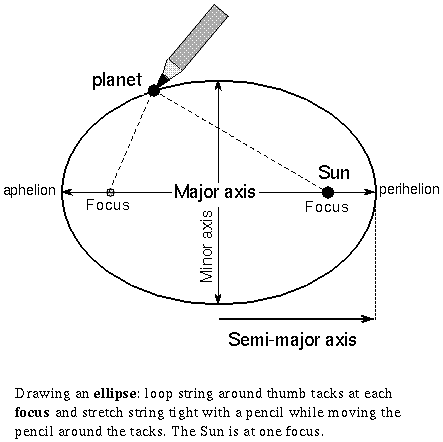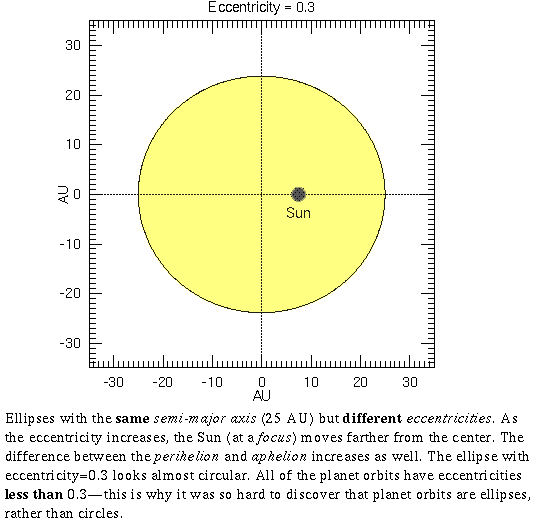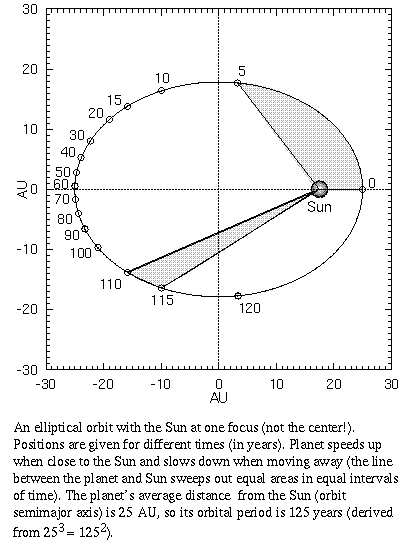## Kepler's Laws of Planetary Motion

#### Chapter index in this window —   — Chapter index in separate window

This material (including images) is copyrighted!. See my copyright notice for fair use practices. Select the photographs to display the original source in another window.Johaness Kepler (lived 1571--1630 C.E.) was hired by Tycho Brahe to work out the mathematical details of Tycho's version of the geocentric universe. Kepler was a religious individualist. He did not go along with the Roman Catholic Church or the Lutherans. He had an ardent mystical neoplatonic faith. He wanted to work with the best observational data available because he felt that even the most elegant, mathematically-harmonious theories must match reality. Kepler was motivated by his faith in God to try to discover God's plan in the universe---to ``read the mind of God.'' Kepler shared the Greek view that mathematics was the language of God. He knew that all previous models were inaccurate, so he believed that other scientists had not yet ``read the mind of God.''

Since an infinite number of models are possible (see Plato's Instrumentalism above), he had to choose one as a starting point. Although he was hired by Tycho to work on Tycho's geocentric model, Kepler did not believe in either Tycho's model or Ptolemy's model (he thought Ptolemy's model was mathematically ugly). His neoplatonic faith led him to choose Copernicus' heliocentric model over his employer's model

Kepler tried to refine Copernicus' model. After years of failure, he was finally convinced with great reluctance of an revolutionary idea: God uses a different mathematical shape than the circle. This idea went against the 2,000 year-old Pythagorean paradigm of the perfect shape being a circle! Kepler had a hard time convincing himself that planet orbits are not circles and his contemporaries, including the great scientist Galileo, disagreed with Kepler's conclusion. He discovered that planetary orbits are ellipses with the Sun at one focus. This is now known as Kepler's 1st law.An ellipse is a squashed circle that can be drawn by punching two thumb tacks into some paper, looping a string around the tacks, stretching the string with a pencil, and moving the pencil around the tacks while keeping the string taut. The figure traced out is an ellipse and the thumb tacks are at the two foci of the ellipse. An oval shape (like an egg) is not an ellipse: an oval tapers at only one end, but an ellipse is tapered at both ends (Kepler had tried oval shapes but he found they did not work).

There are some terms I will use frequently in the rest of this book that are used in discussing any sort of orbit. Here is a list of definitions:

1. Major axis---the length of the longest dimension of an ellipse.
2. Semi-major axis---one half of the major axis and equal to the distance from the center of the ellipse to one end of the ellipse. It is also the average distance of a planet from the Sun at one focus.
3. Minor axis---the length of the shortest dimension of an ellipse.
4. Perihelion---point on a planet's orbit that is closest to the Sun. It is on the major axis.
5. Aphelion---point on a planet orbit that is farthest from the Sun. It is on the major axis directly opposite the perihelion point. The aphelion + perihelion = the major axis. The semi-major axis then, is the average of the aphelion and perihelion distances.
6. Focus---one of two special points along the major axis such that the distance between it and any point on the ellipse + the distance between the other focus and the same point on the ellipse is always the same value. The Sun is at one of the two foci (nothing is at the other one). The Sun is NOT at the center of the orbit!

As the foci are moved farther apart from each other, the ellipse becomes more eccentric (skinnier). See the figure below. A circle is a special form of an ellipse that has the two foci at the same point (the center of the ellipse).

7. The eccentricity (e) of an ellipse is a number that quantifies how elongated the ellipse is. It equals 1 - (perihelion)/(semi-major axis). Circles have an eccentricity = 0; very long and skinny ellipses have an eccentricity close to 1 (a straight line has an eccentricity = 1). The skinniness an ellipse is specified by the semi-minor axis. It equals the semi-major axis × Sqrt[(1 - e2)].

Planet orbits have small eccentricities (nearly circular orbits) which is why astronomers before Kepler thought the orbits were exactly circular. This slight error in the orbit shape accumulated into a large error in planet positions after a few hundred years. Only very accurate and precise observations can show the elliptical character of the orbits. Tycho's observations, therefore, played a key role in Kepler's discovery and is an example of a fundamental breakthrough in our understanding of the universe being possible only from greatly improved observations of the universe.Most comet orbits have large eccentricities (some are so eccentric that the aphelion is around 100,000 AU while the perihelion is less than 1 AU!). The figure above illustrates how the shape of an ellipse depends on the semi-major axis and the eccentricity. The eccentricity of the ellipses increases from top left to bottom left in a counter-clockwise direction in the figure but the semi-major axis remains the same. Notice where the Sun is for each of the orbits. As the eccentricity increases, the Sun's position is closer to one side of the elliptical orbit, but the semi-major axis remains the same.

To account for the planets' motion (particularly Mars') among the stars, Kepler found that the planets must move around the Sun at a variable speed. When the planet is close to perihelion, it moves quickly; when it is close to aphelion, it moves slowly. This was another break with the Pythagorean paradigm of uniform motion! Kepler discovered another rule of planet orbits: a line between the planet and the Sun sweeps out equal areas in equal times. This is now known as Kepler's 2nd law.

Later, scientists found that this is a consequence of the conservation of angular momentum. The angular momentum of a planet is a measure of the amount of orbital motion it has and does NOT change as the planet orbits the Sun. It equals the (planet mass) × (planet's transverse speed) × (distance from the Sun). The transverse speed is the amount of the planet's orbital velocity that is in the direction perpendicular to the line between the planet and the Sun. If the distance decreases, then the speed must increase to compensate; if the distance increases, then the speed decreases (a planet's mass does not change).

Finally, after several more years of calculations, Kepler found a simple, elegant equation relating the distance of a planet from the Sun to how long it takes to orbit the Sun (the planet's sidereal period). (One planet's sidereal period/another planet's sidereal period)2 = (one planet's average distance from Sun/another planet's average distance from Sun)3. Recall that the semi-major axis is the average distance from the Sun (average of perihelion and aphelion). If you compare the planets to the Earth (with an orbital period = 1 year and a distance = 1 A.U.), then you get a very simple relation: (a planet's sidereal period in years)2 = (semi-major axis of its orbit in A.U.)3. This is now known as Kepler's 3rd law. A review of exponents and square roots is available in the mathematics review appendix.

For example, Mars' orbit has a semi-major axis of 1.52 A.U., so 1.523 = 3.51 and this equals 1.872. The number 1.87 is the number of years it takes Mars to go around the Sun. This simple mathematical equation explained all of the observations throughout history and proved to Kepler that the heliocentric system is real. Actually, the first two laws were sufficient, but the third law was very important for Isaac Newton and is used today to determine the masses of many different types of celestial objects. Kepler's third law has many uses in astronomy! Although Kepler derived these laws for the motions of the planets around the Sun, they are found to be true for any object orbiting any other object. The fundamental nature of these rules and their wide applicability is why they are considered ``laws'' of nature.

One final note about Kepler's Third Law: when you plug in different numbers for the semi-major axis (distance), you will see that the corresponding orbit period (time) number gets bigger faster than the corresponding distance number. An orbit twice as big in semi-major axis will have an orbital period more than twice as long; an orbit three times as big in distance will have an orbital period more than three times as long, etc. That's a handy rule of thumb to use to see if your calculation "makes sense", i.e., a check to see if you entered the right exponent powers for the distance and period: period is squared while distance is cubed.Select the image to show an animation of Kepler's 3 laws.

A nice java applet for Kepler's laws is available on the web (select the link to view it in another window).

The UNL Astronomy Education program's Planetary Orbit Simulator allows you to manipulate the various parameters in Kepler's laws to understand their effect on planetary orbits (link will appear in a new window).

### Vocabulary

angular momentum aphelion eccentricity
ellipse focus Kepler's 1st law
Kepler's 2nd law Kepler's 3rd law perihelion
semi-major axis

### Review Questions

1. What shape are planet orbits and where is the Sun with respect to the orbit?
2. What happens to a planet's orbital speed as it approaches its farthest point from the Sun and as it approaches its closest point? How is it related to angular momentum?
3. How were Kepler's laws of planetary motion revolutionary or a radical break from earlier descriptions of planetary motion?
4. A moon's closest distance from a planet is 300,000 km and its farthest distance is 500,000 km. What is the semi-major axis of its elliptical orbit?
5. How will the semi-minor axis compare with the semi-major axis for an ellipse with eccentricity = 0.1, 0.5, 0.8, 0.99? Find the value of (semi-minor/semi-major) for each of the eccentricities.
6. How will the perihelion compare with the aphelion for an ellipse with eccentricity = 0.1, 0.5, 0.8, 0.99? Find the value of (perihelion/aphelion) for each of the eccentricities. [Hint: using the relation that the perihelion + aphelion = 2× semi-major axis and a little algebra, you can find that (perihelion/aphelion) = (1-e)/(1+e).]
7. How is the average distance between a planet and the Sun related to the planet's orbit period?
8. Which planet has a shorter period---one with a large average distance, or one with a small average distance?
9. What is the semi-major axis of an asteroid orbiting the Sun with a period of 64 years? (Kepler's third law works for any object orbiting the Sun.)

#### Go to Astronomy Notes home

last updated: October 1, 2019